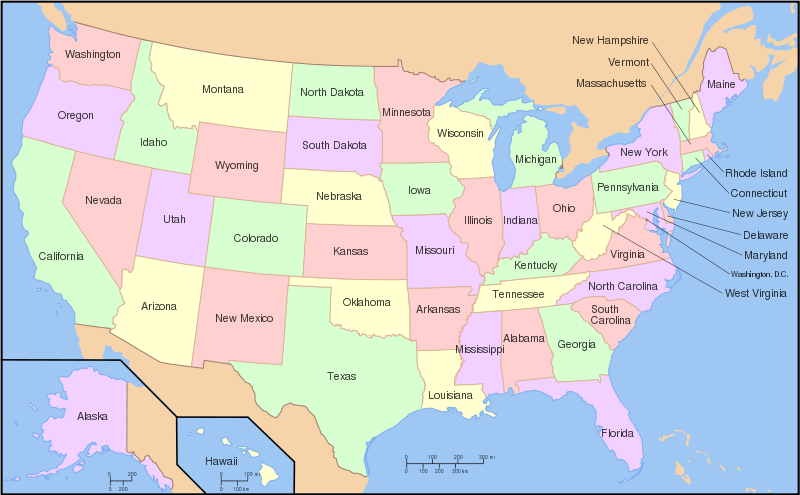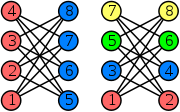# CS 3110 Recitation 22 Graph algorithms

## Graph coloring algorithms

Given an undirected graph, a graph coloring is an assignment of labels traditionally called "colors" to each vertex. A graph coloring must have a special property: given two adjacent vertices, i.e., such that there exists an edge between them, they must not share the same color. The origin of the problem comes from the problem of coloring a map, where two countries (or states) must have different colors when they are adjacent. Here is a map of the United States colored with only 4 colors:Below is a coloring of the Peterson graph using only three colors:Graph colorings have a number of applications:

• Scheduling algorithms: imagine having a set of jobs to do, and a number of workers, you need to assign each worker a job during some time slot (for simplification, assume each job requires one time slot). Jobs can be scheduled in any order, but pairs of jobs may be in conflict in the sense that they may not be assigned to the same time slot, for example because they both rely on a shared resource. The corresponding graph contains a vertex for every job and an edge for every conflicting pair of jobs. If you have an unlimited number of workers, the chromatic number of the graph is exactly the optimal time to finish all jobs without conflicts.
• Register allocation: a compiler is a computer programs that transforms source code from a high-level language (such as C, Java or OCaml) to machine code. This is usually done is several steps, and one of the last steps consists in allocating registers to the most frequently used values of the program, while putting the other ones in memory. We can model this as a graph coloring problem: the compiler constructs an interference graph, where vertices are symbolic registers and an edge connects two nodes if they are needed at the same time. If the graph can be colored with k colors then the variables can be stored in k registers.
• Pattern matching also has applications in graph coloring

In this section we will see how to color a graph with k colors. We say that a graph is k-colorable if and only if it can be colored using k or less colors.

### 2-colorability

There is a simple algorithm for determining whether a graph is 2-colorable and assigning colors to its vertices: do a breadth-first search, assigning "red" to the first layer, "blue" to the second layer, "red" to the third layer, etc. Then go over all the edges and check whether the two endpoints of this edge have different colors. This algorithm is O(|V|+|E|) and the last step ensures its correctness.

### k-colorability for k>2

For k>2 however, the problem is much more difficult. For those interested in complexity theory, it can be shown that deciding whether a given graph is k-colorable for k>2 is an NP-complete problem. The first algorithm that can be thought of is brute-force search: consider every possible assignments of colors to vertices, and check whether there are correct. This, of course, is very expensive, on the order of O((n+1)!), and impractical. Therefore we have to think of a better algorithm.

### A greedy algorithm for finding a non-optimal coloring

Here we will present an algorithm called greedy coloring for coloring a graph. In general, the algorithm does not give the lowest k for which there exists a k-coloring, but tries to find a reasonable coloring while still being reasonably expensive. This is known as an approximation algorithm: we don't find the best solution, but we still find some reasonable solution.

The algorithm is as follows: Consider the vertices in a specific order v1,…,vn and assign to vi the smallest available color not used by vi’s neighbours among v1,…,vi − 1, adding a fresh color if needed. This algorithm finds a reasonable coloring and is O(|V|+|E|).

The problem is that it does not find the optimal k for which the graph is k-colorable. In particular, in some pathological cases, k can be as high as n/2 when the optimal k would be 2. This is true with the following graph (which can be extended to any even number of vertices), where the left ordering leads to a coloring using only 2 colors, and the right ordering leads to 4 colors:### The five color theorem, the four color theorem

A planar graph is a graph which can be embedded in the plane, i.e., it can be drawn on the plane in such a way that its edges intersect only at their endpoints. For a long time, it has been known that any planar graph is 5-colorable, this is known as the five color theorem; the proof is usually done by contradiction and can be found on wikipedia.

For a long time, a conjecture was that any planar graph was 4-colorable, since all the known planar graphs (and maps) had been four-colorable. The conjecture was proposed in 1852, and finally proven in 1976 by Kenneth Appel and Wolfgang Haken using a proof by computer (K. Appel and W. Haken, ‘Every map is four colourable’, Bulletin of the American Mathematical Society 82 (1976), 711–12). The proof was also formalized in the Coq theorem prover by Georges Gonthier.

## PageRank

PageRank is an algorithm first used by the Google search engine which tries to rank how important a web page is by giving it a score (or page rank). Described by Google, "PageRank relies on the uniquely democratic nature of the web by using its vast link structure as an indicator of an individual page's value. In essence, Google interprets a link from page A to page B as a vote, by page A, for page B. But, Google looks at more than the sheer volume of votes, or links a page receives; it also analyzes the page that casts the vote. Votes cast by pages that are themselves "important" weigh more heavily and help to make other pages "important"."

First of all, the web can be seen as very big graph, with the vertices being the web pages, and the edges being the links between web pages. The first idea is that the more a web page is linked to, the more valuable it is. Therefore the idea is to have in-degree in the web graph as a measure of a page's "value". The second idea is that one might want to weight the incoming links proportional to the value of the referring pages. This is now a recursive problem, which is not easily solveable.

### A simplified algorithm

PageRank can be seen as a probability distribution used to represent the likelihood that a person (usually called the surfer) randomly clicking on links will arrive at any particular page. Here, we will take this assumptions, and so the different scores will be number between 0 and 1, adding up to 1. The algorithm presented here is an iterative algorithm and it is simplified.

Let us work out an example on four pages A, B, C and D. Since there are four pages, we initialize the values of the PR (PageRanks) to 0.25 each. Let us say pages B, C and D point to A, and that B has 2 outgoing edges, C one and D three. A simplistic view would compute the new PR(A) as PR(A)=PR(B)+PR(C)+PR(D). However the pageranks have to be normalized by the number of outgoing edges of each page, and we get PR(A)=PR(B)/2+PR(C)/2+PR(D)/3. More generally, if L(P) is the number of outgoing links for page P, then PR(A)=PR(B)/L(B)+PR(C)/L(C)+PR(D)/L(D). In the most general case, the new PR(P) is the sum of all the PR(Q)/L(Q), for all Q pointing to P.

Actually, the imaginary surfer has to stop clicking at some point. In the PageRank theory, the probability that the surfer stops clicking is called the dumping factor and is denoted 1-d (d being the probability that he continues to click. That is, the new PageRank of A is: PR(A)=1-d+d(PR(B)/L(B)+PR(C)/L(C)+PR(D)/L(D)+...).

It can be shown that this algorithm converges. In the limit it can be solved as an eigenvector problem on a matrix representation of the graph, but we won't go into details about that.